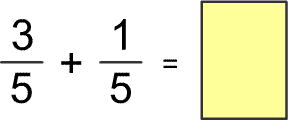Skill 26A Add Like Fractions (same denominator) A "like fraction" simply means that both fractions have the same denominators (bottom number), while "unlike fractions" have different denominators. Of course, adding like fractions is easy - just add the numerators (top number) and the denominator stays the same. So, the answer to this would be 4/5. What is the sum to this fraction addition problem. 1.